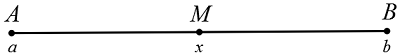# SAT LinesIn the diagram above, points $A$ and $B$ have coordinates $a$ and $b,$ such that $b>a.$ If $M$ is the midpoint of segment $\overline{AB},$ and $M$ has the coordinate $x,$ all of the following are true EXCEPT:

(A) $\ \ AM = \frac{1}{2}AB$
(B) $\ \ MB = b-x$
(C) $\ \ \overline{AM} \cong \overline{MB}$
(D) $\ \ 2x = a+b$
(E) $\ \ x = 2b-a$

×